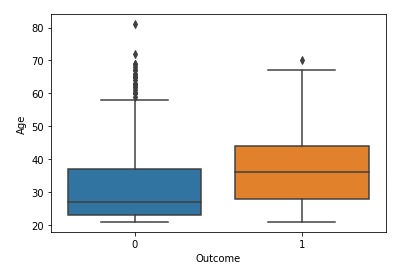#### Visualization of healthcare data with two python libraries

In this article, we will discuss some basic visualization with matplotlib and seaborn library. Both libraries are well known in the data science and analytics community.

• Matplotlib: It is very useful to plot basic plotting functionality with a customizable approach. It is very much comfortable with pandas and numpy. It also helps to plot multiple figures.
• Seaborn: It is also a very powerful tool for visualization and more comfortable with a pandas data frame. It provides beautiful themes to the plot. It provides multiple figures but sometimes leads to OOM (Out Of Memory) problems.

Some examples of visualization with matplotlib and seaborn are shown below:

#### Matplotlib Library

To make visualization we need to import data with the help of the pandas’ library.

`import pandas as pd`

`#reading the CSV file with read_csv in pandasdf = pd.read_csv('healthcare.csv')`

To get a view of data

`df.head()`
• Boxplot

It is used to see the quartiles of the as descriptive analysis.

`import matplotlib.pyplot as plt#To check outliers with the help of box plot for column in df:    if df[column].dtype in ['int64', 'float64']:        plt.figure()        df.boxplot(column = [column])`
• Histogram of all features in one figure

The histogram is a visualization analysis to see the distribution of the data.

`df.hist()`
• Plotting single histogram of one column feature
`#plotting one histogram plt.hist(df['BMI'])`
• Plotting single scatter plot of two-column features

Scatter plots are used to see the relationship between two variables.

`#comparision two features on custom scatter plotx = df['Age']y = df['Glucose']`
`plt.scatter(x,y)plt.xlabel('Age')plt.ylabel('Glucose')plt.title('Age vs Glucose')plt.show(`
• Bar plot

Bas plots are used to see the values of categorical variables with their counts.

`plt.bar(x,y)`
• Plotting scatter plot of all features as subplots.
`ax[0,0].scatter(x = df['Age'], y = df['BMI'])ax[0,0].set_xlabel("Age")ax[0,0].set_ylabel("BMI")ax[0,1].scatter(x = df['Age'], y = df['SkinThickness'])ax[0,1].set_xlabel("Age")ax[0,1].set_ylabel("SkinThickness")ax[0,2].scatter(x = df['Age'], y = df['DiabetesPedigreeFunction'])ax[0,2].set_xlabel("Age")ax[0,2].set_ylabel("DiabetesPedigreeFunction")ax[1,0].scatter(x = df['Age'], y = df['Insulin'])ax[1,0].set_xlabel("Age")ax[1,0].set_ylabel("Insulin")ax[1,1].scatter(x = df['Age'], y = df['BloodPressure'])ax[1,1].set_xlabel("Age")ax[1,1].set_ylabel("BloodPressure")ax[1,2].scatter(x = df['Age'], y = df['Pregnancies'])ax[1,2].set_xlabel("Age")ax[1,2].set_ylabel("Pregnancies")ax[2,0].scatter(x = df['Age'], y = df['Glucose'])ax[2,0].set_xlabel("Age")ax[2,0].set_ylabel("Glucose")plt.show()`

#### Seaborn Library

First, import the library

`import seaborn as sns`
• Plotting a joint plot of the histogram and scatter plot.
`sns.jointplot(x=x, y=y, data=df, size=5)`
• Bloxplot in seaborn
`sns.boxplot(x="Outcome", y="Age", data=df)`The box plot with the seaborn library. A photo by Author
• Boxplot with data points
`sns.boxplot(x="Outcome", y="Age", data=df)`
`sns.stripplot(x="Outcome", y="Age", data=df, jitter=True,              edgecolor="gray")`
• Violin plot

It is used to see the probability density of the data and similar to the box plot.

`sns.violinplot(x="Outcome", y="Age", data=df, size = 6)`
• Scatter plot with seaborn
`a = sns.scatterplot(x = "Age", y = "Glucose", hue = "Outcome", data                    = df)`
• Pairplot

It is used to see the relationship between variables of whole data in one figure.

`aa=sns.pairplot(df)`
• Plotting distribution curve with histogram
`plt.figure(figsize=(12,12))plt.subplot(3,3,1)sns.distplot(df.Pregnancies)plt.subplot(3,3,2)sns.distplot(df.Glucose)plt.subplot(3,3,3)sns.distplot(df.BloodPressure)plt.subplot(3,3,4)sns.distplot(df.SkinThickness)plt.subplot(3,3,5)sns.distplot(df.BMI)plt.subplot(3,3,6)sns.distplot(df.DiabetesPedigreeFunction)`
• Count plot

It is used to see the values or count of the categorical features.

`sns.countplot(x="Pregnancies",data=df)`

#### Conclusion:

The visualization is a good method to observe the relationship between features and get some insights from them. There are many plots and graphs but in this article, we discussed some of them.

Understand List as Big O and Comprehension with Python Examples

I hope you like the article. Reach me on my LinkedIn and twitter.

### Recommended ArticlesMatplotlib and Seaborn Visualization with Python was originally published in Analytics Vidhya on Medium, where people are continuing the conversation by highlighting and responding to this story.